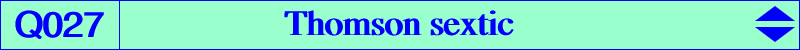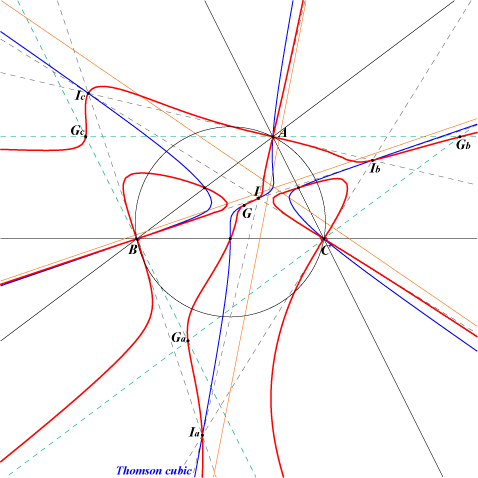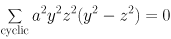X(1), X(2), X(174), X(188), X(366), X(5374) excenters midpoints of ABC vertices of the antimedial triangle points at infinity of the sidelines of ABC points at infinity of the Thomson cubic harmonic associates of X(174), X(188), X(366) and actually of any of its points since it is quadratic in x, y, z. extraversions of X(174), X(188) and actually of any of its weak points since it is a strong curve CPCC or H-cevian points, see Table 11Q027 is the locus of point P such that the anticevian and cevian triangles of P are orthologic. Q027 has six real asymptotes : three are the sidelines of the antimedial triangle and the three other are parallel to those of the Thomson cubic. A, B, C are double points with tangents the bisectors. The tangents at the midpoints of ABC are the medians. Q027 is the locus of the fixed points of the isoconjugations having their pole on the Thomson cubic. In other words, Q027 contains the square roots of the points of the Thomson cubic. Thus, Q027 is invariant under harmonic association and also under extraversion. In particular, it contains the 8 square roots of the foci of the Steiner in-ellipse. These points lie on the diagonal conics passing through G, the vertices of the antimedial triangle which are tangent at G to the axes of this ellipse. See also here for other properties (Angel Montesdeoca, 2019-08-26, in Spanish). See Q058, a very similar sextic.